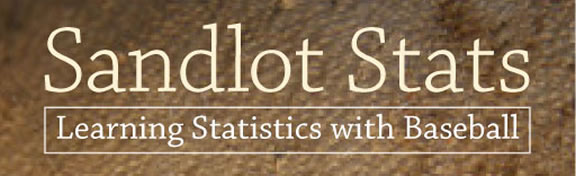an innovative textbook that explains the mathematical underpinnings of baseball so that students can understand the world of statistics and probability - The Johns Hopkins University Press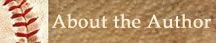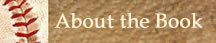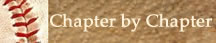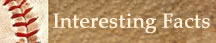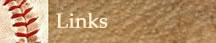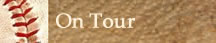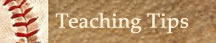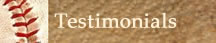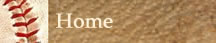Get Sandlot Stats on your phone. Scan in the code above. Chapter By Chapter This chapter outline describes what you can find inside of Sandlot Stats. Chapter 1 introduces the terms and definitions for the concepts presented in any statistics course. An actual “hot dog study” done by the Los Angeles Dodgers is used to illustrate these concepts. Examples, in and out of the world of baseball, are provided for each of the concepts introduced. Chapter 2 introduces the standard techniques used in descriptive statistics. These techniques are applied to one quantitative variable. Standard statistical measures are used to find the middle of the data and how and how spread out the data is.  Graphs are used to give a shape to the data. The normal curve is the most common shape. The properties common to all normal curves are presented. Chapter 3 introduces the descriptive measures specific to baseball such as batting average, on-base percentage, slugging percentage, and home run rate. Chapter 4 uses the techniques of descriptive statistics to compare two quantitative data sets. In particular, a comparison of the batting performances of Henry Aaron and Barry Bonds is made. Chapter 5 introduces regression and correlation analysis. Relationships between various baseball descriptive measures are explored. Regression analysis is used to establish linear relationships between various baseball statistics and the runs scored by a player or by a team. Correlation analysis is used to find which baseball statistics are the best predictor of runs scored by a player or by a team. Chapter 6 applies the concepts of descriptive statistics to one or more qualitative variables. Contingency tables and special graphs are used to find a relationship between two qualitative variables. The concept of when two variables are independent is introduced. Chapter 7 introduces the concept of probability. Three types of probabilities are defined. They are classical probability, relative frequency probability, and subjective probability. The Fundamental Counting Principle, permutations, and combinations are defined. A contingency table for Aaron and Bonds is displayed, and simple and compound probabilities are computed. Independent and mutually exclusive events are defined. Conditional probability is introduced and used to compare qualitative variables. Side-by-side and stacked bar graphs are used to observe relationships between qualitative variables. Probability disks are constructed for Aaron and Bonds. Simulations are done using both physical and theoretical models. Chapter 8 introduces the concepts involved in sports betting. The concept of odds is introduced. The relationship between odds and probability is explored. The expected gain and loss resulting from making a bet are explained. A discussion of how a casino profits from sports betting is presented. Examples demonstrating both casino betting and sports betting are presented. A discussion is made of how to approach sports betting. Chapter 9 relates the standard descriptive measures used in any statistics course to the descriptive measures specific to baseball. Chapter 10 uses the baseball descriptive statistics evaluated in the previous chapters to draw some conclusions on which player, Aaron or Bonds, was a better hitter. Chapter 11 looks at discrete probability distributions. The idea of a theoretical mathematical model is introduced. The binomial distribution and the geometric distribution are defined. Baseball situations are modeled using both of these distributions. Probabilities using the geometric and binomial model are calculated. Chapter 12 looks at continuous probability distributions. The most important continuous distribution is the normal distribution. Probabilities are calculated using the normal curve. Chapter 13 looks at the concept of a sampling distribution. Sampling distributions for sample means and for sample proportions are studied. The role of a sampling distribution as a bridge to inferential statistics is explained. Chapters 14 and 15 introduce the two major techniques used in statistical inference. They are confidence intervals and hypothesis testing. Chapter 14 introduces the inferential technique of confidence intervals. A new continuous distribution, called the t-distribution, is introduced. This chapter differentiates between a sample baseball statistic and a population baseball parameter. Confidence intervals are used to estimate both the population batting average and population on-base percentage for Aaron and Bonds. The term level of confidence is introduced. Chapter 15 introduces the inferential technique of hypothesis testing. Three methods of hypothesis testing are presented. They are classical hypothesis testing, the p-value approach, and the confidence interval approach. An eight-step method for classical hypothesis testing is presented. Hypothesis testing for one population mean and for one population proportion is illustrated. These techniques are used to explore such issues as whether either Aaron or Bonds was a true career.300 hitter. The term statistical significance is explained. The concept of level of significance is introduced. The next two chapters are involved with two research studies in baseball. The information covered in the first 15 chapters is applied to these baseball research questions. Chapter 16 studies different baseball batting streaks. Much of the chapter involves Joe DiMaggio’s 56-game hitting streak. The 84-game on-base streak of Ted Williams and other lesser-known streaks are also discussed. The purpose of this chapter is to apply the concepts of probability and statistics, from the previous 15 chapters, to analyze the probability of these streaks happening. The probability of various players achieving each of these streaks is examined. Chapter 17 looks at the fabulous baseball feat of batting .400 for a season. The last .400 hitter was Ted Williams, who accomplished this feat in 1941. All .400 hitters, since 1913, are presented. An attempt is made to present the baseball characteristics that seem to be necessary for a player to hit .400. The likelihood of this feat being duplicated in the future is analyzed. Chapter 18 is the concluding chapter. In this chapter, the following questions are analyzed: What are the greatest hitting feats of all time? Which hitting feat will be the hardest to duplicate? Who are the top hitters in various baseball eras? Who are the top 10 hitters of all time? In order to decide on the top 10 hitters of all time, a scoring system is developed that is based on the statistics covered in prior chapters in this book. Chapter 18 concludes with a final set of chapter problems that involve the concepts covered in many of the prior chapters. The final problem asks the student to finalize their decision on whether their chosen player should be elected into the Hall of Fame.

Sandlot Stats: Learning Statistics with Baseball by Stanley Rothman is published by The Johns Hopkins University Press